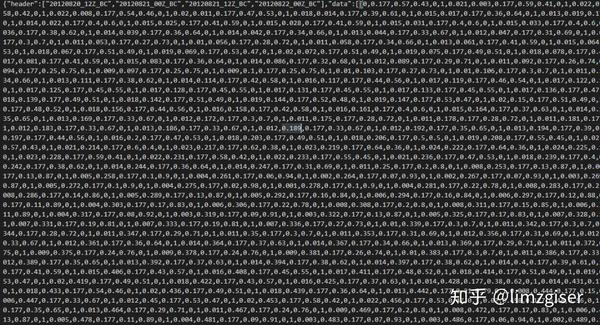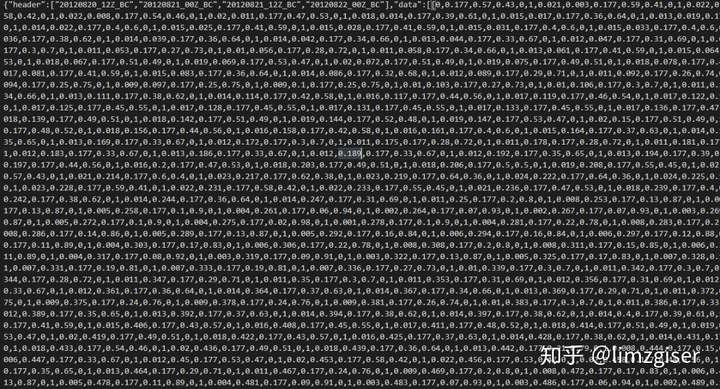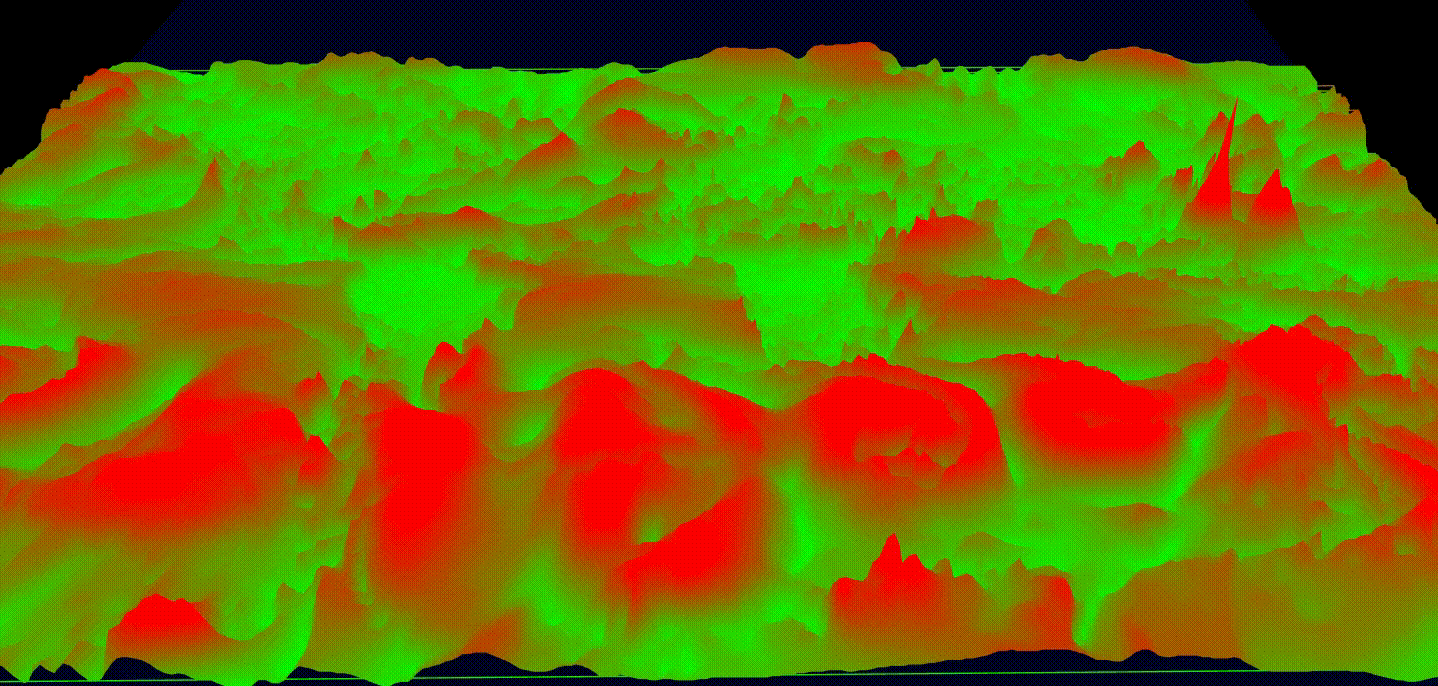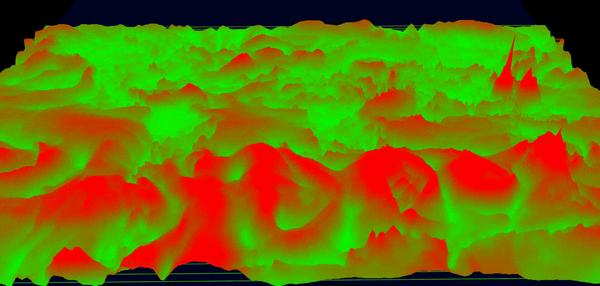337次阅读

## 二、获取气象数据（风力风向）

webgl-wind 提到了数据获取和处理的方式。我尝试了一波，软件下载和环境配置。失败了！！！！windows环境下好像不行，我电脑的配置，让我放弃了。https://github.com/linghuam/ocean-weather​github.com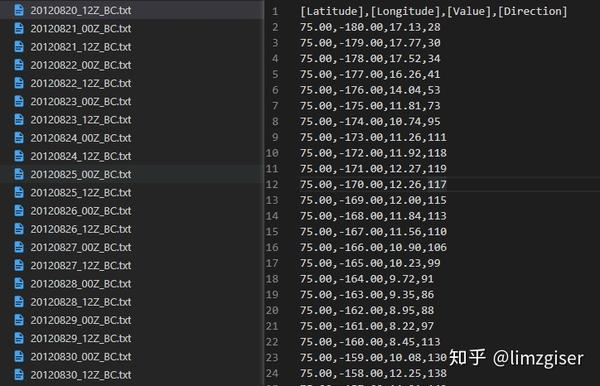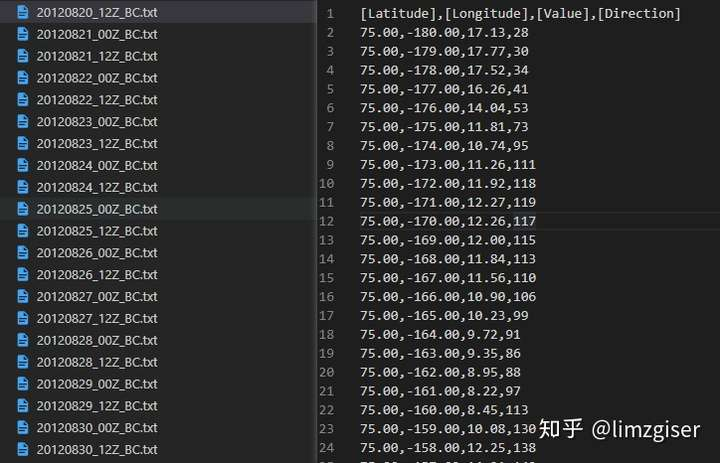[Latitude],[Longitude],[Value],[Direction]

[经度]，[纬度]，[风力]，[风向]

## 三、mapboxgl + webgl 基础

WebGL 理论基础​webglfundamentals.org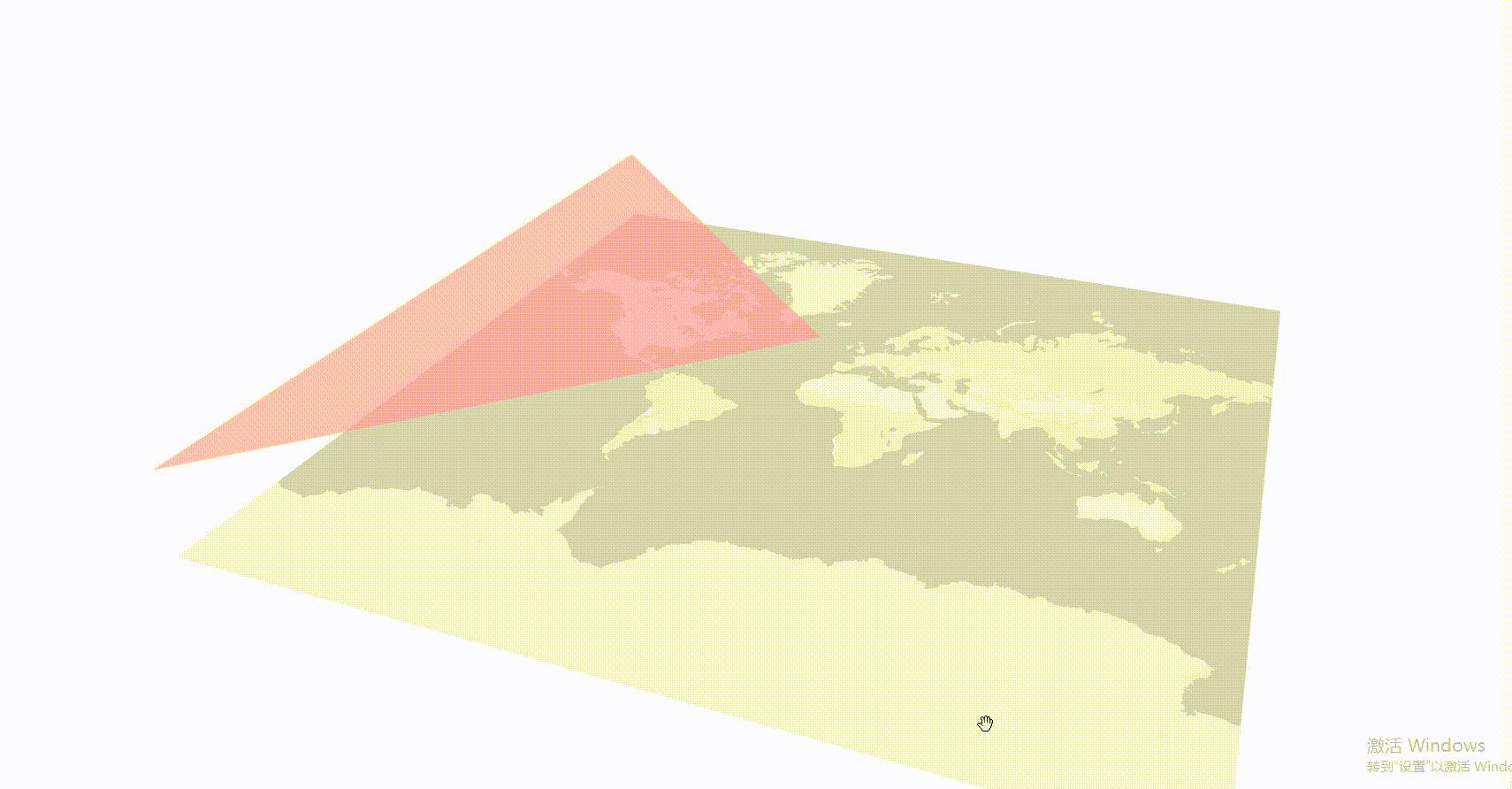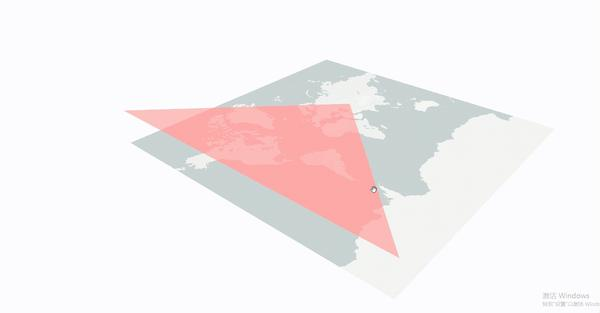mapboxgl提供自定义图层，自行编写着色程序，渲染几何数据。

```{
id: 'layerid',
type: 'custom',
},
render: function (gl, matrix) {
}
};```

render：帧循环函数，渲染每一帧时候调用。gl是地图上下文对象，matrix是相机矩阵，它将球面的墨卡托坐标投影到gl坐标。

WebGL在电脑的GPU中运行。因此需要使用能够在GPU上运行的代码。 这样的代码需要提供成对的方法，一个是顶点着色器，另一个是片元着色器，他们一对组成了着色程序。其中，顶点着色器用来计算顶点位置，片元着色器负责对几何图行进行光珊话处理，为每个像素着色。

1、顶点着色器

```  uniform mat4 u_matrix;// 矩阵，视口矩阵
uniform float  anim;  // 全局变量（在案例中作用是用来修改顶点高度）
attribute vec2 a_pos; // 属性，将会从缓冲中获取数据,表示空间 x,y
void main() {
// gl_Position为webgl内置变量
// 将(x,y,z,1)组成的四维向量，通过u_matrix矩阵变换为gl坐标
gl_Position = u_matrix * vec4(a_pos , anim , 1.0);
}```

2、片元着色器

``` void main() {
// 片元（填充像素被着色为红色半透明）
gl_FragColor = vec4(1.0, 0.0, 0.0, 0.5);
}```

3、创建着色程序

```// 创建顶点着色器
// 提供顶点数据源——定点着色器代码字符串
// 编译，生成顶点着色器
// 创建片元着色器
// 提供片元着色器数据源——片元着色器代码字符串
// 编译片元着色器
// 创建着色程序-并链接顶点着色器和片元着色器
this.program = gl.createProgram();

4、向着色程序传数据

```// 从顶点着色器中获取 a_pos 属性所在的位置
this.aPos = gl.getAttribLocation(this.program, 'a_pos');
// 从顶点着色器中获取 anim 属性所在的位置
this.unifromAnim = gl.getUniformLocation(this.program, "anim");
// 三角形顶点坐标，将wgs84经纬度坐标转墨卡托投影坐标，
// 投影后加过真个地球坐标0到1
// 左上为[0,0],右下为[0，1]
//{x: 0, y: 0.0016379147860542769, z: 0}
var p1= mapboxgl.MercatorCoordinate.fromLngLat({  lng: -180.00,lat: 85  });
// {x: 0.5, y: 0.5, z: 0}
var p2= mapboxgl.MercatorCoordinate.fromLngLat({  lng: 0,lat: 0});
// {x: 0, y: 0.9983620852139461, z: 0}
var p3 = mapboxgl.MercatorCoordinate.fromLngLat({ lng: -180.00,  lat: -85 });
// 创建buffer存储数据，着色器中的 a_pos 属性将从buffer里面获取数据
this.buffer = gl.createBuffer();
// 绑定buffer
gl.bindBuffer(gl.ARRAY_BUFFER, this.buffer);
// 绑定数据
gl.bufferData(gl.ARRAY_BUFFER,new Float32Array([
p1.x,
p1.y,
p2.x,
p2.y,
p3 .x,
p3 .y
]),gl.STATIC_DRAW );```

5、渲染数据

```// 使用色程序，着色程序可能有多个，绘制数据前指定使用哪个着色程序
gl.useProgram(this.program);
// 给视口矩阵赋值
gl.uniformMatrix4fv(gl.getUniformLocation(this.program, 'u_matrix'), false,  matrix);
animTime += 0.002;
animTime = animTime % 0.2;
// 给anim变量赋值
gl.uniform1f(this.unifromAnim, animTime);
// 使用缓冲前要先绑定
gl.bindBuffer(gl.ARRAY_BUFFER, this.buffer);
//启用 a_pos 属性
gl.enableVertexAttribArray(this.aPos);
// 指定从缓冲中读取数据的策略
gl.vertexAttribPointer(this.aPos, 2, gl.FLOAT, false, 0, 0);
// 启用颜色混合，大概意思就是就是把某一像素位置原来的颜色和将要画上去的颜色，通过某种方式混在一起，从而实现特殊的效果
gl.enable(gl.BLEND);
// 指定颜色混合方式
gl.blendFunc(gl.SRC_ALPHA, gl.ONE_MINUS_SRC_ALPHA);
// 绘制图元，三个点，一个三角形
gl.drawArrays(gl.TRIANGLE_STRIP, 0, 3);```

6、结果## 四、风力数据绘制

1、离散点

```精度    维度   风力
75.00,-180.00,17.13
75.00,-179.00,17.77
75.00,-178.00,17.52```

```// 定点
0                                 // x
0.17729912095967093               // y
0.5725490196078431                // r
0.42745098039215684               // g
0                                 // b
1                                 // w
---- 分隔
0.002777777777777778
0.17729912095967093
0.592156862745098
0.40784313725490196
0
1
---- 分隔
0.005555555555555556
0.17729912095967093
0.5843137254901961
0.41568627450980394
0
1```

```// csv文件字符串，转一维数组
function tof32Array(csvstr) {
let tmparr = csvstr.split(/[\n]/);
let res = [];
for (let i = 0; i < tmparr.length; i += 1) {
let item = tmparr[i];
let tmpitem = item.split(',');
let nor = mapboxgl.MercatorCoordinate.fromLngLat({
lng: tmpitem,
lat: tmpitem,
});
let tmp = [ nor.x, nor.y, ...calcColor(tmpitem)];
res.push(...tmp);
}
return res;
}
// 根据风力计算颜色
function calcColor(value) {
var aa = d3.rgb(255, 0, 0); //红色
var bb = d3.rgb(0, 255, 0); //绿色
var compute = d3.interpolate(aa, bb);
var linear = d3.scale.linear()
.domain([0, 30])
.range([1, 0]);
let tmpres = compute(linear(value));
let rgb = tmpres.split(',');
let r = rgb.split('(');
let g = rgb;
let b = rgb.split(')');
return [r / 255.0, g / 255.0, b / 255.0, 1.0]
}```

```// 顶点着色器
uniform   mat4  u_matrix;
attribute vec2  a_pos;
attribute vec4  inColor;
varying   vec4  outColor;
void main() {
outColor     = inColor;
gl_Position  = u_matrix * vec4(a_pos,0 , 1.0);
gl_PointSize = 2.0;
}
// 片元着色器
precision  lowp  float;
varying    vec4  outColor;
void main() {
gl_FragColor = outColor;
}```

```// 点坐标
gl.vertexAttribPointer(this.aPos, 2, gl.FLOAT, false, 4 * 6, 0);
// 点颜色
gl.vertexAttribPointer(this.inColor, 4, gl.FLOAT, false, 4 * 6, 4 * 2);
// 绘制图元--点
gl.drawArrays(gl.POINTS, 0, pointLen);```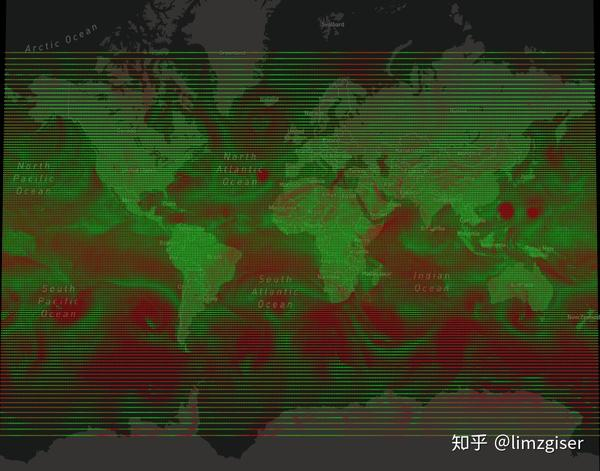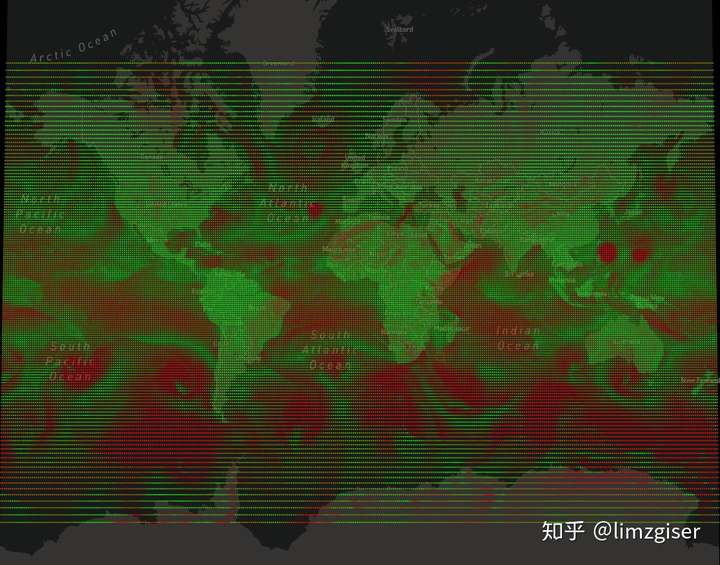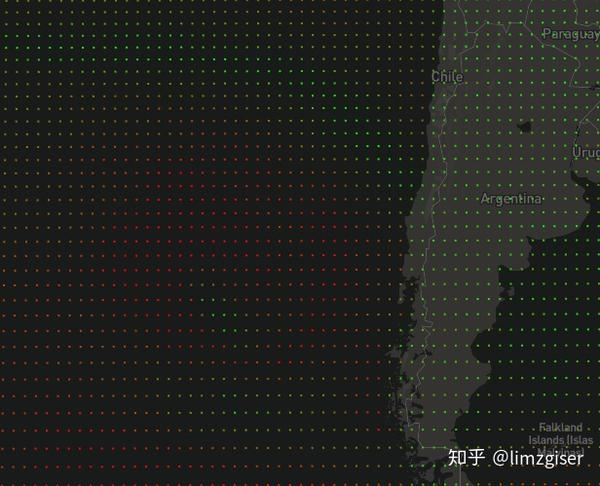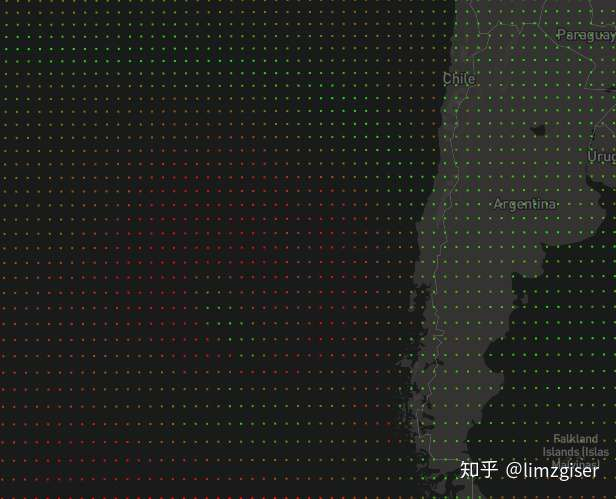2、离散点+高度

```0: 0                            // x
1: 0.17729912095967093          // y
2: 0.5725490196078431           // r
3: 0.42745098039215684          // g
4: 0                            // b
5: 1                            // w
6: 0.04894285714285714          // 风力/350
7: 0.002777777777777778
8: 0.17729912095967093
9: 0.592156862745098
10: 0.40784313725490196
11: 0
12: 1
13: 0.05077142857142857
14: 0.005555555555555556
15: 0.17729912095967093
16: 0.5843137254901961
17: 0.41568627450980394
18: 0
19: 1
20: 0.050057142857142856
21: 0.008333333333333333```
```// 点坐标
gl.vertexAttribPointer(this.aPos, 2, gl.FLOAT, false, 4 * 7, 0);
// 颜色
gl.vertexAttribPointer(this.inColor, 4, gl.FLOAT, false, 4 * 7, 4 * 2);
//定点高度
gl.vertexAttribPointer(this.pointH, 1, gl.FLOAT, false, 4 * 7, 4 * 6);```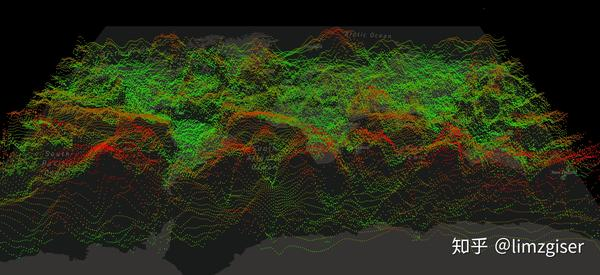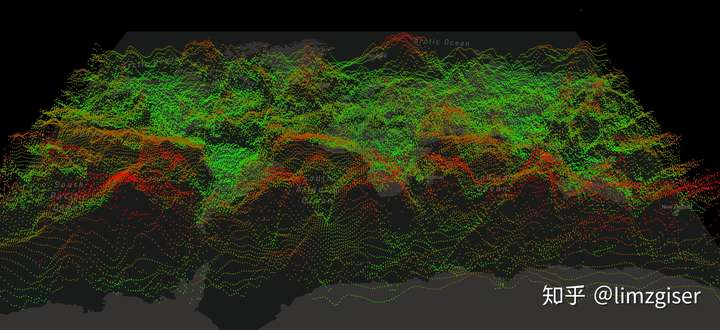3、插值

webgl在绘制一个三角形，指定三个顶点颜色后，webgl在进行光栅化时，会根据三个顶点的颜色进行插值计算每个像素的颜色。之前我们绘制顶点， 已经根据风力计算出来每个顶点的颜色，只要我们把绘制图元类型改成三角形（TRIANGLES），webgl在光栅话的时候，会做插值处理。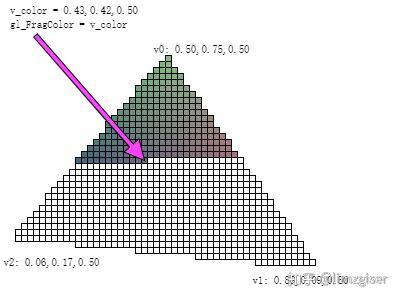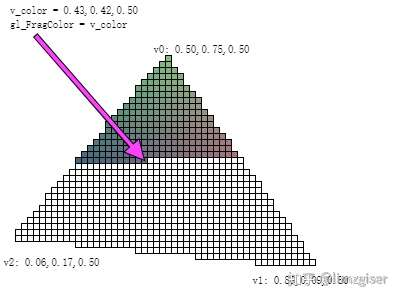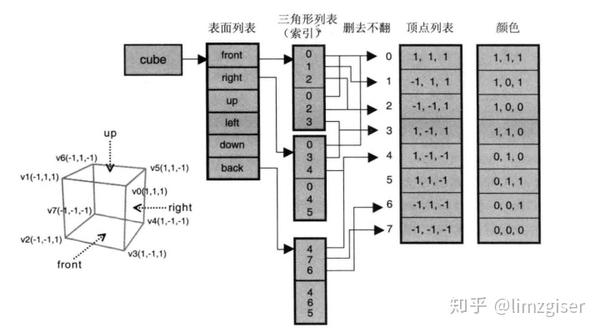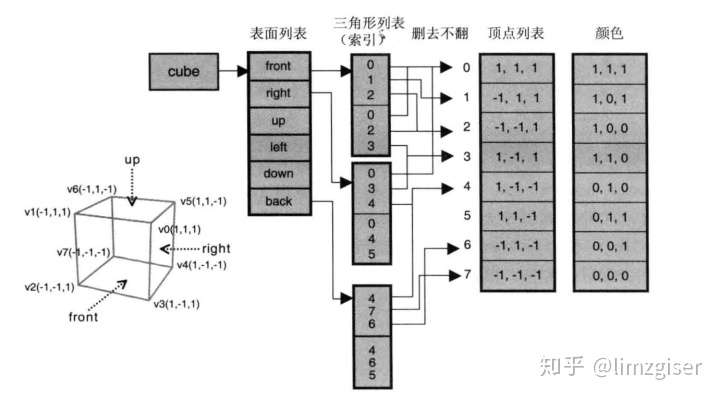```// 计算顶点索引
function getElementIndex() {
var arr = [];
for (var i = 0; i < 150; i++) {
for (var j = 0; j < 360; j++) {
var x = j + 361 * i;
var y = x + 1;
var z = x + 361;
var arr1 = [x, y, z, y, z, ++z];
arr.push(...arr1);
}
}
return arr;
}```

` gl.drawElements(gl.LINE_STRIP, indexEle.length, gl.UNSIGNED_SHORT, 0);`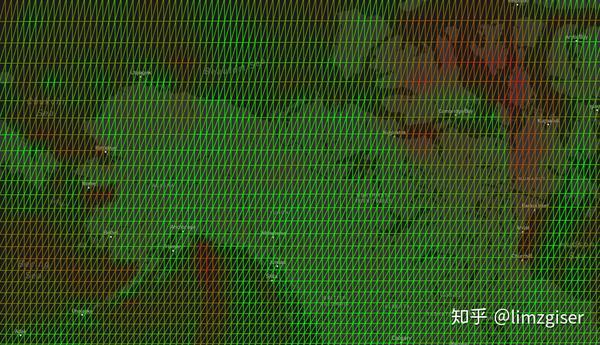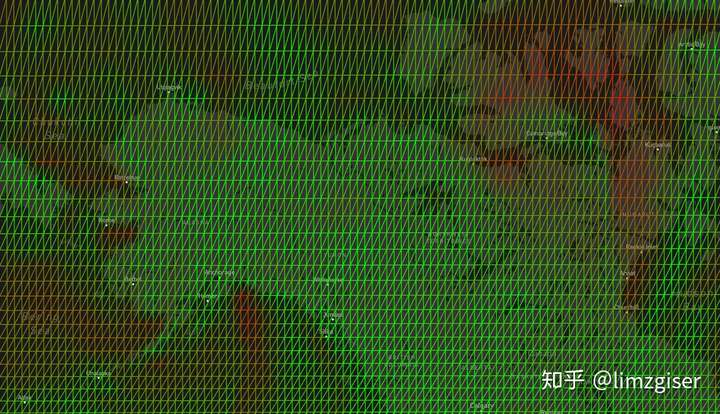`gl.drawElements(gl.TRIANGLES, indexEle.length, gl.UNSIGNED_SHORT, 0);`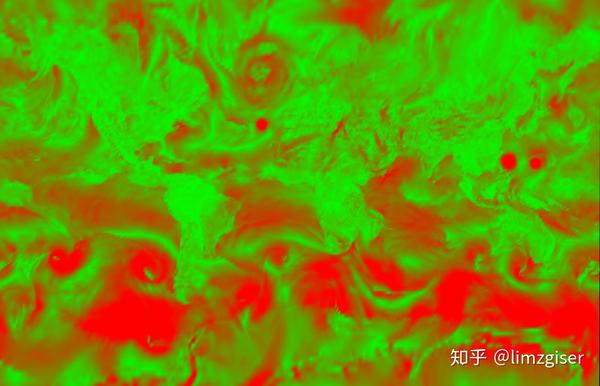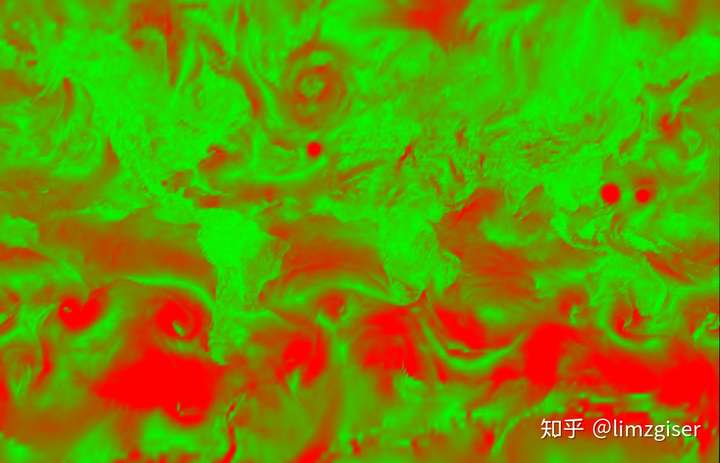`gl_Position =   u_matrix * vec4(a_pos,pointH , 1.0); `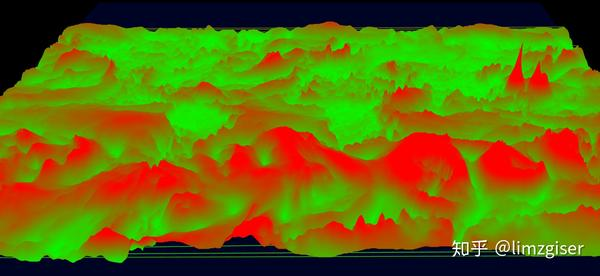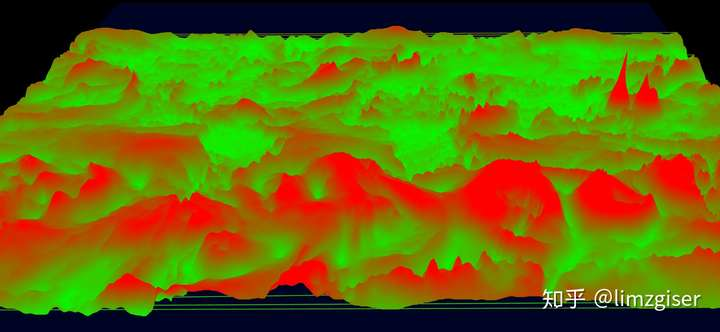3、多日全球风力动画